# Nonlinear numerical analysis of convective-radiative fin using MLPG method

Nonlinear numerical analysis of convective-radiative fin using MLPG method

Rajul Garg*Harishchandra Thakur Brajesh Tripathi

Department of Mechanical Engineering, School of Engineering, Gautam Buddha University, Greater Noida, U.P., India

Department of Mechanical Engineering, Rajasthan Technical University, Kota, Rajasthan, India

Corresponding Author Email:
garg.rajul2010@
Page:
721-729
|
DOI:
https://doi.org/10.18280/ijht.350405
|
Accepted:
|
Published:
31 December 2019
| Citation

OPEN ACCESS

Abstract:

A mathematical model describing nonlinear and transient heat transfer through a straight insulated tip fin with temperature-dependent heat transfer coefficient has been addressed by the meshless local Petrov- Galekin (MLPG) method. Moving least square approximants are used to approximate the unknown function of temperature T(x) with Th (x).These approximants are constructed by using a linear basis, a weight function and a set of non-constant coefficients. Essential boundary conditions are imposed by penalty method. An iterative predictor-corrector scheme is used to handle nonlinearity and two-level q method for temporal discretization. The accuracy of MLPG method is verified by comparing the results for the simplified versions of the present model with an exact analytical solution. Once the accuracy of MLPG method is established, the method is used to generate results for the complex heat transfer problems formulated here. Temperature variation along the fin length over the discrete time range till the attainment of steady state, under convective and convective-radiative environment has been demonstrated.

Keywords:

Convective- radiative Fin, MLPG Method, Penalty method, Nonlinear Fin Analysis, Transient Analysis

1. Introduction

Heat transfer is a natural phenomenon but under certain conditions it becomes inevitable to transfer heat or remove the excess heat from the hot primary surface at a faster rate. Heat transfer can be enhanced either by using highly conductive material or by increasing the heat transfer coefficient, which may require the installation of artificial heat transfer systems like pumps or fans etc. Another way of enhancing the heat transfer is the increasing of surface area. But virtually, the material, operating and geometric properties can be enhanced up to a certain limit and hence the objective of necessary amount of heat removal turns down. In such situations, the alternative is to increase the surface area by attaching extension to the primary hot surface. This extension is called extended surface or fin.

The primary objective of fin is to enhance the heat transfer rate per unit area between the base surface and its convective, radiative and convective- radiative environment. Today, due to increasing demand of high performance parametric values but at the same time compactness in heat bearing structures, the extended surfaces or fins of different geometries and material properties are found to be used in wide range of general as well as sophisticated engineering applications which include air conditioning, aerospace, automobile, chemical processing equipments, from large industrial heat exchangers to small systems such as transistors and other electronic components.

Increasing use and the potential development of fins in the wide engineering domains have attracted many authors to concentrate their research on the performance of fins under variable and dynamic conditions. Over the period of time different numerical methods have been employed by the researchers to analyse the behavior of fins. Singh et al.  have used meshless element free Galerkin method; Cole et al.  have employed Green’s functions (GF) in the form of infinite series; Basri et al.  have shown confidence on efficient finite element method and differential quadrature method; Wang et al.  have presented method of superposition and separation variables; Singh et al.  and Sao and Banjare  have used quasi- steady theory; finite volume method has been employed by Lotfi and Belkacem  and Al- Rashed et al. ; Taler and Taler  have presented the coupling of finite volume method- finite element method; B- spline based finite element method has been highlighted by Reddy et al.  and field synergy principle optimization analysis has been conducted by Wei et al.  to analyse the heat transfer problems of fins.

But general engineering applications including heat transfer problems, in actual, are non-linear in nature. This nonlinearity arises due to the reliance of thermal as well as material properties on the temperature or cross- sectional area of the fin. Optimal linearization method has been used by Jordan et al. ; Frobenius expanding series has been employed by Kundu and Das ; homotopy analysis method has been presented by Khani et al.  and Amirkolaei and Ganji ; incremented differential quadrature method has been highlighted by Malekzadeh and Rahideh ; Ganji et al.  and Sobamowo  have used Galerkin method; Moradi and Ahmadikia , Sadri et al. , Ndlovu and Moitsheki , Mosayebidarchech et al. , Ghasemi et al.  and Ganji and Dogonchi  have used differential transformation method; homotopy perturbation method has been presented by Arslanturk , Ganji et al.  and Hoshyar et al. ; Aziz and Bouaziz  have employed method of least squares; Kirchoff’s transformation method has been used by Moitsheki and Rowjee ; lie point symmetry method has been highlighted by Moitsheki and Harley , Mhlongo and Moitsheki , Ali et al.  and Kader et al. ; Hajabdollahi et al.  has presented genetic algorithm; symbolic programming has been used by Fatoorehchi and Abolghasemi ; and Latif et al.  have used symmetry reduction method successfully, to address nonlinear heat transfer problems of fins. Mahmoudi and Mejri  have investigated the effect of variable thermal conductivity and variable refractive index on transient conduction and radiation heat transfer by Lattice Boltzmann method. Sun et al.  have demonstrated the convective-radiative fin with temperature dependent properties.

To the best of authors’ knowledge, meshless local Petrov- Galerkin (MLPG) method has not been used to study the behavior of fins. MLPG method was developed by Atluri and Zhu [39- 40]. Unlike FEM and most other meshfree methods, MLPG method operates on local weak form and performs integration over overlapping simple local domains. This has removed the need of mesh at any stage of analysis. Hence, it is truly a meshfree method.

2. Governing Equation and Mlpg Formulation

One dimensional heat transfer equation is considered. Heat transfer coefficient follows the power law and is temperature-dependent. Other properties are taken as constant. There is no heat generation in the solid. The governing differential equation Ω is given by

$k \frac{\partial^{2} T}{\partial x^{2}}-h(T)\left(\frac{P_{r}}{A_{c}}\right)\left(T-T_{a}\right)-\varepsilon \sigma\left(\frac{P_{r}}{A_{c}}\right)\left(T^{4}-T_{s}^{4}\right)$$=\rho c \frac{\partial T}{\partial t} (1) If, hr= \varepsilon \sigma\left(T^{2}+T_{s}^{2}\right)\left(T+T_{s}\right) then Eq. (1) becomes, k \frac{\partial^{2} T}{\partial x^{2}}-h(T)\left(\frac{P_{r}}{A_{c}}\right)\left(T-T_{a}\right)-h_{r}\left(\frac{P_{r}}{A_{c}}\right)\left(T-T_{s}\right)$$-\rho c \frac{\partial T}{\partial t}=0$      (2)

Initial and boundary conditions:

$T(x, 0)=T_{o}$       on $\Omega$        (3)

$\left\{\begin{array}{l}{T(x, t)=\overline{T}} \\ {q(x, t)=\overline{q}} \\ {q(x, t)=h\left(T_{a}-T\right)}\end{array}\right.$     on $\Gamma_{1}$

on $\Gamma_{2}$

on $\Gamma_{3}$     (4)

where $\Gamma=\Gamma_{1} \cup \Gamma_{2} \cup \Gamma_{3}$  and  $q=k\left(\frac{\partial T}{\partial n}\right)$

MLPG method is based on local weak form. Weighted residual formulation for Eq. (2) in local domain $\Omega_{Q}$ can be expressed as

$\int_{\Omega_{0}} v\left[\begin{array}{c}{k \frac{\partial^{2} T}{\partial x^{2}}-h(T)\left(\frac{P_{r}}{A_{c}}\right)\left(T-T_{a}\right)} \\ {-h_{r}\left(\frac{P_{r}}{A_{c}}\right)\left(T-T_{s}\right)-\rho c \frac{\partial T}{\partial t}}\end{array}\right] d \Omega=0$   (5)

Where, v is the test function. According to Atluri and Shen , the MLPG method is classified into six sub- methods, based on the way the test function is chosen as MLPG 1 (MLS weight function); MLPG 2 (Dirac’s Delta function); MLPG 3 (discrete least sqaures); MLPG 4 (fundamental solution); MLPG 5 (Heaviside unit step function); MLPG 6 (identical to trial function). Although, all the MLPG methods possess higher accuracy, still MLPG 1 is claimed as one of the strongest method to address complicated heat transfer problems as it yields the better results and higher rate of convergence than the established methods [40-47]. Hence MLPG 1 is employed in this study.

Using divergence theorem, Eq. (5) yields the desired weak form as:

$\left[k \frac{\partial T}{\partial n} v\right]_{\Gamma_{Q}}-\int_{\Omega_{Q}}\left[\begin{array}{cc}{k \frac{\partial T}{\partial x} \frac{\partial v}{\partial x}+h(T) v\left(\frac{P_{r}}{A_{c}}\right) T-h(T) v\left(\frac{P_{r}}{A_{c}}\right) T_{a}+} \\ {h_{r} v\left(\frac{P_{r}}{A_{c}}\right) T-h_{r} v\left(\frac{P_{r}}{A_{c}}\right) T_{s}+\rho c \frac{\partial T}{\partial t} v}\end{array}\right] \mathrm{d} \Omega=0$   (6)

Where $\Gamma_{Q}$ is the boundary of the local domain, $\Omega_{O}$ . In case of 1-D problem, boundary integrals turn to be a point value on boundaries. Taking advantage of MLPG method’s flexibility, the test function “v” is selected such that it vanishes at the boundary of local domain. Hence, boundary integral remains non-zero only when local domain intersects the global boundary. The essential boundary conditions are imposed by penalty function method, developed by Zhu and Atluri . Therefore, Eq. (6) can be written as:

$[q v]_{\Gamma_{1 Q}}+[\overline{q v}]_{\Gamma_{20}}+\left[v h(T)\left(T_{a}-T\right)\right]_{\Gamma_{3 Q}}$$-\int_{\Omega_{\varrho}}\left[\begin{array}{c}{k \frac{\partial T}{\partial x} \frac{\partial v}{\partial x}+h(T) v\left(\frac{P_{r}}{A_{c}}\right) T-h(T) v\left(\frac{P_{r}}{A_{c}}\right) T_{a}} \\ {+h_{r} v\left(\frac{P_{r}}{A_{c}}\right) T-h_{r} v\left(\frac{P_{r}}{A_{c}}\right) T_{s}+\rho c \frac{\partial T}{\partial t} v}\end{array}\right] d \Omega$$-\alpha[(T-\overline{T}) v]_{\Gamma_{10}}=0$        (7)

Where $\Gamma_{1 Q}=\Gamma_{1} \cap \Gamma_{Q}, \Gamma_{2 Q}=\Gamma_{2} \cap \Gamma_{Q}, \Gamma_{3 Q}=\Gamma_{3} \cap \Gamma_{\varrho}$ and α is the penalty function parameter = 1 x 1010

The unknown function, T, at any instant of time t, is approximated by moving least square scheme (Lancaster and Salkauskas ) as follows:

$T(\mathbf{x})=\sum_{i=1}^{n_{s}} \Phi_{i} T_{i}=\mathbf{\Phi} \mathbf{T}$       (8)

where $\Phi$ is the vector of meshfree shape functions $\Phi_{i}$ , T represents the vector of nodal parameters Ti at time t and ns is the number of nodes in the support domain at point x. Substituting the approximation (8) in Eq. (7) and performing integration over all local domains corresponding to all field nodes, the semi-discrete system can be obtained as follows:

$\mathbf{C} \mathbf{T}+\mathbf{K} \mathbf{T}=\mathbf{F}$      (9)

When the essential boundary condition is imposed with the penalty method, Eq. (9) can be written in the form

$K_{i j}$ = [$\int_{\Omega_{Q}}\left(\begin{array}{c}{\frac{\partial v_{i}}{\partial x} k \frac{\partial \Phi_{j}}{\partial x}+h(T) v_{i}\left(\frac{P_{r}}{A_{c}}\right) \Phi_{j}} \\ {+h_{r} v_{i}\left(\frac{P_{r}}{A_{c}}\right) \Phi_{j}}\end{array}\right) \mathrm{d} \Omega$$-\left[v_{i} \frac{\partial \Phi}{\partial n}\right]_{\Gamma_{1} \varrho}$$+\left[v_{i} h(T) \Phi_{j}\right]_{\Gamma_{3 Q}}+\left[\alpha v_{i} \Phi_{j}\right]_{\Gamma_{1 \varrho}}$]         (10)

$C_{i j}=\int_{\Omega_{0}} \rho c v_{i} \Phi_{j} \mathrm{d} \Omega$        (11)

$F_{i}=\left[v_{i} \overline{q}\right]_{\Gamma_{2 Q}}+\left[v_{i} h(T) T_{a}\right]_{\Gamma_{3 Q}}+$$\int_{\Omega_{0}}\left(h(T) v_{i}\left(\frac{P_{r}}{A_{c}}\right) T_{a}+h_{r} v_{i}\left(\frac{P_{r}}{A_{c}}\right) T_{s}\right) \mathrm{d} \Omega$$+\left[\alpha v_{i} \overline{T}\right]_{\Gamma_{1} \varrho}$         (12)

Spatial discretization of governing partial differential equation (2) results in a system of semi-discrete ordinary differential equations. Two- level q method for temporal discretization has been used in the present analysis. It can vary between explicit and implicit strategies and results in the algebraic system.

$[\mathbf{C}+\theta \Delta t \mathbf{K}] \mathbf{T}^{n+1}=[\mathbf{C}+(\theta-1) \Delta t \mathbf{K}] \mathbf{T}^{n^{\prime}}+\Delta \boldsymbol{F}$        (13)

where, n’ denotes the time level.

According to Morgan , nonlinear systems can be very complicated, if not impossible, to solve explicitly. The majority of nonlinear analysis of systems of ODEs focuses on whether or not the systems have stable equilibria. The equilibrium characterizes as stable or unstable based on the behavior of solutions whose initial conditions are in the neighborhood of the equilibrium. If solutions near a critical point of a system stay close to the critical point as time approaches infinity, the critical point is assumed to be stable.

An iterative predictor-corrector scheme, based on direct substitution iteration (Lewis and Roberts ) is used to handle nonlinearity in the current work. This scheme is an algorithm that proceeds in two steps. It calculates a rough approximation of the desired quantity in the first step and refines approximation in the next by any other means. It combines the advantages associated with explicit and implicit time schemes and hence provides the stable solution to solve complex nonlinear problems:

Predictor:

$\left[\mathbf{C}\left(\mathbf{X}^{n}\right)+\theta \Delta t \mathbf{A}\left(\mathbf{X}^{n}\right)\right] \mathbf{X}_{*}^{n+1}=$ $\left[\mathbf{C}\left(\mathbf{X}^{n}\right)+(1-\theta) \Delta t \mathbf{A}\left(\mathbf{X}^{n}\right)\right] \mathbf{X}^{n}+\Delta t \mathbf{B}\left(\mathbf{X}^{n}\right)$        (14)

$\left[\mathbf{C}\left(\mathbf{X}_{p}^{\overline{m}}\right)+\theta \Delta t \mathbf{A}\left(\mathbf{X}_{p}^{\overline{m}}\right)\right] \mathbf{X}_{p+1}^{n+1}=$$\left[\mathbf{C}\left(\mathbf{X}_{p}^{\overline{n}}\right)+(1-\theta) \Delta t \mathbf{A}\left(\mathbf{X}_{p}^{\overline{\pi}}\right)\right] \mathbf{X}^{n}+\Delta t \mathbf{B}\left(\mathbf{X}_{p}^{\overline{n}}\right)$        (15)

where p = 0, 1, 2, 3…up to convergence and

$\begin{array}{ll}{\mathbf{X}_{p}^{n}=w \mathbf{X}_{p}^{n+1}+(1-w) \mathbf{X}^{n}} & {0 \leq w \leq 1} \\ {\mathbf{X}_{0}^{n+1}=\mathbf{X}_{*}^{n+1}}\end{array}$        (16)

3. Results and Discussions

Consider a sample problem of one-dimensional fin as shown in the figure 1.

## 1.png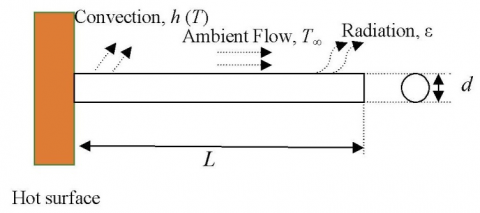Figure 1. Schematic of cylindrical fin

The different parameters used for the transient analysis are listed in Table 1

Table 1. Thermo-geometric parameters of fin

 S. No. Thermo- Geometric Parameters Value of Parameters 1 d 0.02 m 2 L 0.10 m 3 Pr 0.0628 m 4 c 0.48 kJ/kg.0C 5 ρ 7800 kg/m3 6 Ac 1.57 x 10-4 m2 7 k 12 W/m.0C 8 h (temperature dependent) 9.0 ∆T0.175 W/m2.0C 9 h (constant) 22 W/m2.0C 10 Ta 300C 11 Tb 2000C 12 Tinit 2000C 13 ∆t 10 sec

3.1 Validation of results

Table 2. Validation of results

 Dimensionless Temperature $\left(T-T_{a}\right) /\left(T_{b}-T_{a}\right)$ x, m MLPG FVM (Holman, ) Exact 0.02 0.6952 0.6968 0.6949 0.04 0.4943 0.4964 0.4935 0.06 0.3667 0.369 0.3657 0.08 0.2936 0.2959 0.2925 0.10 0.2640 0.2665 0.2630 Heat Transfer Through Fin Qfin, W 11.9700 12.0820 11.8740

In this section, the problem is solved with constant value of heat transfer coefficient and without considering the effects of radiation. The results obtained by MLPG method are compared with exact solution of the problem and also with the result obtained by using finite volume method . Results obtained by using MLPG method are found to be very close to the exact solution as shown in Table 2. Results of MLPG method depend on various parameters and a set of parameters are also searched to obtain best possible results. The one dimensional problem domain is equally distributed in 21 local domains represented by 21 nodes. Extent of quadrature domain is finally taken as, αQ = 1.66 and support domain as, αS =2.5. The penalty method is employed to impose the essential boundary conditions. The maximum relative error with exact solution is found to be 0.38%. Heat transfer through fin is also calculated and shown in Table 2.

Fin equation is solved initially for steady state condition using MLPG method. Parameters are chosen as discussed in previous section. Fin is investigated under three different conditions -- under the condition of constant heat transfer coefficient, under the condition of variable heat transfer coefficient which is a function of temperature difference between the fin surface and the surroundings and finally under the combine effects of convection and radiation. For radiation, emissivity of the fin surface is assumed to be one.

## 2.pngFigure 2. Variation of temperature along the fin under steady state condition

Variation of temperature under all three conditions is plotted in Fig. 2. Two plots represent constant and variable heat transfer coefficients respectively which are coinciding initially but part away as moving towards the tip of the fin. This is due to the fact that variable heat transfer coefficient is a function of temperature difference between the fin surface and surrounding medium. This difference in temperature is higher near the base of the fin and results a very closer value to the constant value of heat transfer coefficient in this region. Temperature difference decreases along the length of fin and so will be the heat transfer coefficient. The plot of combined convection and radiation starts with steepest slope and reaches at the lowest value of the temperature. This is due to increased value of heat transfer along the fin due to radiation.

3.2.1 Effect of emissivity

Actual fin surfaces are always not black and the emissivity of the real surfaces lies between 0 to 1. Different values of emissivity will yield different values of heat transfer in radiation. Temperature distribution for different values of surface emissivity is plotted in Fig. 3. Fin with black surface attains lowest temperature at its tip showing maximum amount of heat transfer.

## 3.png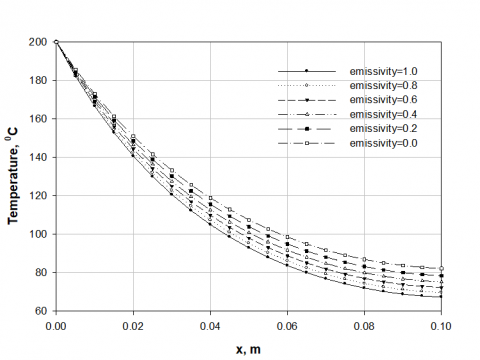Figure 3. Effect of emissivity on variation of temperature along the fin

3.3 Transient analysis

After steady state analysis, the transient analysis is performed to understand fin response with respect to time. Three cases taken in of the previous section are considered and compared. Emissivity is taken as 1. Figure 4 shows variation of temperature along the fin at different instant of time for three cases. Due to greater value of temperature difference between fin surface and surrounding fluid, initially both the convection curves are very close to each other and radiation effect is very high. Higher value of radiation heat transfer results in a big gap between the convection and radiation curves. This gap reduces with time as shown in corresponding plots as temperature difference reduces with time.

Figure 5 shows temperature-time history of four different points on the fin. Temperature of all the points decrease at a faster rate initially, slows down thereafter and finally tending to reach a constant value showing to be near to steady state. Effect of radiation can be seen in all the plots as points are cooling down at faster rate. As we move away from the hot base (from a to d), minimum temperature which is going to be prevailed on that under steady state reduces as expected.

## 4a.png(a)

## 4b.png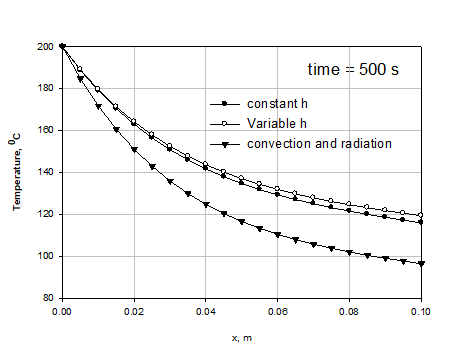(b)

## 4c.png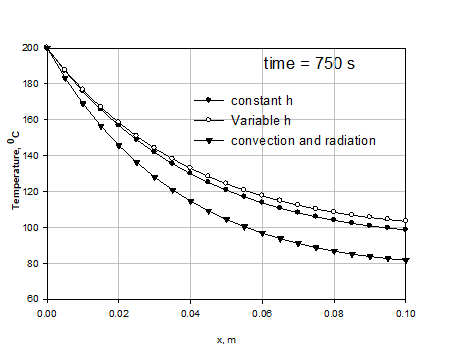(c)

## 4d.png(d)

Figure 4. Variation of temperature at different times along the fin length in three cases

## 5a.png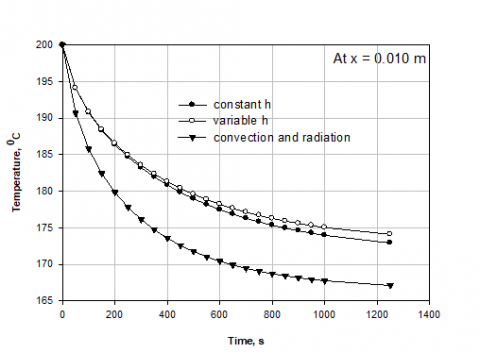(a)

## 5b.png(b)

## 5c.png(c)

## 5d.png(d)

Figure 5. Temperature-time history at different positions along the fin length in three cases

3.4 Performance analysis of the fin

Heat Transfer through fin in steady state is given by,

$Q_{f i n}=\int_{0}^{L} h\left(T-T_{a}\right) P d x$       (17)

or it can also be evaluated by the expression

$Q_{f i n}=-k A\left.\frac{d T}{d x}\right|_{\mathrm{x}=0}=-k A \sum_{i=1}^{n}\left(\frac{d \Phi_{i}}{d x} T_{i}\right)_{x=0}$       (18)

Eq. (17) is based on the calculation of total convective heat transfer from the fin surface while Eq. (18) calculates the rate of conduction on the fin base. For the condition of convection with radiation the value of convective heat transfer coefficient is modified to represent combined effects.

Efficiency of the fin is given by,

$\eta_{f i n}=Q_{f i n} / Q_{\max , f i n}$ and$Q_{\max , f i n}=\int_{0}^{L} h\left(T_{b}-T_{a}\right) P d x$        (19)

$Q_{\mathrm{max}, \text {fin}}$  corresponds to the condition when the entire fin is maintained at its base temperature.

Effectiveness of the fin is given by,

$\eta_{f i n}=Q_{f i n} / Q_{\text {witiout} \text { fin}}$ and $Q_{\text {without}, f i n}=h\left(T_{b}-T_{a}\right) A$        (20)

$Q_{\text {without}, f i n}$ is the amount of heat transfer from that area on the base plate where the fin is applied. Heat transfer coefficient is placed for corresponding conditions.

Table 3 shows performance of the fin under different conditions. Heat transfer in case of constant ‘h’ is more than that of the case of variable ‘h’ as constant value is wrong assumption of higher value of ‘h’ throughout the fin surface. When radiation is introduced higher heat transfer is observed depending on the value of surface emissivity. A maximum difference of 20% in heat transfer rate is observed once radiation is taken into account. As emissivity reduces heat transfer rate also reduces while other performances increase. Corresponding values of effective heat transfer coefficient plays its role in denominator to decide these parameters.

Table 3. Performance of fin

 Only convection Condition Qfin(W) Efficiency(%) Effectiveness h= constant 11.97 50.72 10.14 h =variable 11.44 58.90 11.32 Convection and Radiation Emissivity=1.0 14.52 54.62 10.92 Emissivity=0.8 13.96 55.39 11.07 Emissivity=0.6 13.38 56.20 11.24 Emissivity=0.4 12.77 57.05 11.41 Emissivity=0.2 12.12 57.95 11.99
4. Conclusion

Numerical simulation of convective-radiative fin is performed using MLPG method. The MLPG method is a truly meshfree method as it does not need mesh either at the stage of interpolation or at the integration. Fins are very commonly used to increase the rate of heat transfer but it is very essential to predict results with high accuracy. MLPG method can solve nonlinear fin problems and predict accurate solution. Although, MLPG method consumes more computational time than the established methods like FEM, FVM and FDM, in solving complex 1-D heat transfer problems, still with the advent of new generation computers (higher processing rate) this limitation can be eliminated upto a greater extent.

Nomenclature
 d Fin diameter [m] L Fin length [m] Pr Fin perimeter [m] c Specific heat [kJ/kg.0C] K Thermal conductivity [W/m.0C] Ac Cross- sectional area [m2] h Heat transfer coefficient [W/m2.0C] Ta Ambient temperature [0C] Ts Surface temperature [0C] Tb Base temperature [0C] Tinit Initial temperature [0C] ∆t Time step [sec.] t Time [sec.] hr Radiative heat transfer coefficient [W/m2.0C] $\overline{T}$ Specified temperature on essential boundary [0C] $\overline{q}$ Heat flux at natural boundary [W/m2] n Outward unit normal to the boundary Greek symbol α Extent of domains ρ Density of the material [kg/m3] ε Emissivity σ Stephen- Boltzmann constant (5.67 x 10-8 W/m2-K) Ω Global domain Γ Boundary of global domain Γ1 Essential boundary Γ2 Normal boundary Γ3 Convective boundary
References

 Singh I.V., Sandeep K., Prakash R. (2003). Heat transfer analysis of two-dimensional fins using a meshless element free Galerkin method, Numerical Heat Transfer, Part A, Vol. 44, pp. 73-84. DOI: 10.1080/10407780390122826

 Cole K.D., Tarawneh C., Wilson B. (2009). Analysis of flux-base fins for estimation of heat transfer coefficient, International Journal of Heat and Mass Transfer, Vol. 52, Issue 1-2 (January 15, 2009), pp. 92–99. DOI: 10.1016/j.ijheatmasstransfer.2008.05.031

 Basri S., Fakir M.M., Mustapha F., Majid D.L.A., Jaafar A.A. (2009). Heat distribution in rectangular fins using efficient finite element and differential quadrature methods, Engineering, Vol. 1, pp. 151-160. DOI: 10.4236/eng.2009.13018

 Wang P.Y., Kuo G.C., Hu Y.H., Liaw W.L. (2012). Transient temperature solutions of a cylindrical fin with lateral heat loss, Wseas Transactions on Mathematics, Vol. 11, Issue 10, pp. 918-925.

 Singh T., Shrivastava S., Ber H.S. (2013). Analysis of     unsteady heat conduction through short fin with     applicability of quasi theory, Int. J. Mech. Eng. &     Rob. Res., Vol. 2, No. 1, pp. 269-283.

 Sao A.K., Banjare Y.P. (2014). Analysis of thermal characteristics of transient heat conduction through long fin and comparison with exact fin theory and quasi steady theory, International Journal of Emerging Technology and Advanced Engineering. Vol. 4, No. 11, pp. 157-166.

 Lotfi B., Belkacem A. (2014). Numerical method     for optimum performance of fin profiles, International     Journal of Current Engineering and Technology, Vol.     4, No. 6, pp. 3990-3998.

 Al-Rashed A.A.A.A., Kolsi L., Oztop H.F., Abu- Hamdeh N., Borjini M.N. (2017). Natural convection and entropy production in a cubic cavity heated via pin- fins heat sinks, International Journal of Heat and Technology, Vol. 35, No. 1, pp. 109-115. DOI: 10.18280/ijht.350115

 Taler D., Taler J. (2014). Steady-state and transient heat transfer through fins of complex geometry, Archives of Thermodynamics. Vol. 35, No. 2, pp. 117-133. DOI: 10.2478/aoter-2014-0017

 Reddy C.S., Reddy Y.R., Srikanth P. (2014). Application of B–spline based FEM to one-dimensional problems, International Journal of Current Engineering and Technology, Spe. 3,     pp. 137-140.

 Wei Z., Haifang L., Xiaoze D., Yongping Y., Lei S. (2016). Numerical and experimental research on performance of single- row finned tubes in air-cooled power plants, International Journal of Heat and Technology, Vol. 34, No. 1, pp. 137-142. DOI: 10.18280/ijht.340120

 Jordan A., Khaldi S., Benmouna M., Borucki A. (1987). Study of non-linear heat transfer problems, Revue Phys. Appl., Vol. 22, pp. 101-105. DOI: 10.1051/rphysap:01987002201010100

 Kundu B., Das P.K. (2002). Performance analysis and optimization of straight taper fins with variable heat transfer coefficient, International Journal of Heat and Mass Transfer, Vol. 45, pp. 4739- 4751. DOI: 10.1016/S0017-9310(02)00189-8

 Khani F., Ahmadzadeh Raji M., Hamedi Nejad H. (2009). Analytical solutions and efficiency of the nonlinear fin problem with temperature-dependent thermal conductivity and heat transfer coefficient, Commun Nonlinear Sci Numer Simulat, Vol. 14, pp. 3327-3338. DOI: 10.1016/j.cnsns.2009.01.012

 Amirkolei S.R. Ganji D.D. (2014). Thermal performance of a trapezoidal and rectangular profiles fin with temperature - dependent heat transfer coefficient, thermal conductivity and emissivity, Indian J. Sci. Res., Vol. 1, No. 2, pp. 223-229.

 Malekzadeh P., Rahideh H. (2009). Two-dimensional nonlinear transient heat transfer analysis of variable section pin fins, Energy Conversion and Management, Vol. 50, pp. 916-922. DOI: 10.1016/j.enconman.2008.12.025

 Ganji D.D., Raghoshay M., Rahimi M., Jafari M. (2010). Numerical investigation of fin efficiency and temperature distribution of conductive, convective and radiative straight fins, IJRRAS, pp. 230-237. DOI: 10.1002/htj.20341

 Sobamowo M.G. (2016). Thermal analysis of longitudinal fin with temperature dependent properties and internal heat generation using Galerkin’s method of weighted residual, Applied Thermal Engineering, Vol. 99, pp. 1316-1330. DOI: 10.1016/j.applthermaleng.2015.11.076

 Moradi A., Ahmadikia H. (2010). Analytical solution for different profiles of fin with temperature dependent thermal conductivity, Mathematical Problems in Engineering, Vol. 2010, pp. 1-15. DOI: 10.1155/2010/568263

 Sadri S., Raveshi M.R., Amiri S. (2011). Efficiency analysis of straight fin with variable heat transfer coefficient and thermal conductivity, Journal of Mechanical Science and Technology, Vol. 26, No. 4, pp. 1283-1290. DOI: 10.1007/s12206-012-0202-4

 Ndlovu P.L., Moitsheki R.J. (2013). Analytical solutions for steady heat transfer in longitudinal fins with temperature dependent properties, Mathematical Problems in Engineering, Vol. 2013, pp. 1-14. DOI: 10.1155/2013/273052

 Mosayebidorcheh S., Ganji D.D., Farzinpoor M. (2014). Approximate solution of the nonlinear heat transfer equation of a fin with the power-law temperature- dependent thermal conductivity and heat     transfer coefficient, Propulsion and Power Research, Vol. 3, No. 1, pp. 41-47. DOI: 10.1016/j.jppr.2014.01.005

 Ghasemi S.E., Hatami M., Ganji D.D. (2014). Thermal analysis of convective fin with temperature-dependent thermal conductivity and heat generation, Case Studies in Thermal Engineering, Vol. 4, pp. 1-8. DOI: 10.1016/j.csite.2014.05.002

 Ganji D.D., Dogonchi A.S. (2014). Analytical investigation of convective heat transfer of a longitudinal fin with temperature- dependent thermal conductivity, heat transfer coefficient and heat generation, International Journal of Physical Sciences, Vol. 9, No. 21, pp. 466-474. DOI: 10.1080/10407782.2015.1081043

 Arslanturk C. (2010). Optimization of straight fins with step change in thickness and variable thermal conductivity by homotopy perturbation method, J. of Thermal Science and Technology, Vol. 10, pp. 09-19. DOI: 10.4236/eng.2009.13018

 Ganji D.D., Ganji Z.Z., Ganji H.D. (2011). Determination of temperature distribution for annular fins with temperature dependent thermal conductivity by HPM, Thermal Science, Vol. 15, Suppl. 1, pp. S111-S115. DOI: 10.2298/TSCI11S1111G

 Hoshyar H.A., Rahimipetroudi I., Ganji D.D., Majidian A.R. (2015). Thermal performance of porous fins with temperature-dependent heat generation via the homotopy perturbation method and collocation method, Journal of Applied Mathematics and Computational Mechanics, Vol. 14, No. 4, pp. 53-65. DOI: 10.17512/jamcm.2015.4.06

 Aziz A., Bouaziz M.N. (2011). A least squares method for a longitudinal fin with temperature dependent internal heat generation and thermal conductivity, Energy Conversion and Management, Vol. 52, pp. 2876-2882. DOI: 10.1016/j.enconman.2011.04.003

 Moitsheki R.J., Rowjee A. (2011). Steady heat transfer through a two- dimensional rectangular straight fin, Mathematical Problems in Engineering, Vol. 2011, pp. 1-13. DOI: 10.1155/2011/826819

 Moitsheki R.J., Harley C. (2011). Transient heat transfer in longitudinal fins of various profiles with temperature-dependent thermal conductivity and heat transfer coefficient, PRAMANA, Vol. 77, No. 3, pp. 519–532. DOI: 10.1007/s12043-011-0172-6

 Mhlongo M.D., Moitsheki R.J. (2014). Some exact solutions of nonlinear fin problem for steady heat transfer in longitudinal fin with different profiles, Advances in Mathematical Physics, Vol. 2014, pp. 1-16. DOI: 10.1155/2014/947160

 Ali S.A., Bokhari A.H., Zaman F.D. (2014). A Lie symmetry classification of a nonlinear fin equation in cylindrical coordinates, Abstract and Applied     Analysis, Vol. 2014, pp. 1-10. DOI: 10.1155/2014/ 527410

 Kader A.H.A., Latif M.S.A., Nour H.M. (2016). General exact solution of the fin problem with variable thermal conductivity, Propulsion and Power Research, Vol. 5, No. 1, pp. 63-69. DOI: 10.1016/j.jppr.2016.01.007

 Hajabdollahi F., Rafsanjani H.H., Hajabdollahi Z., Hamidi Y. (2012). Multi- objective optimization of pin fin to determine the optimal fin geometry using genetic algorithm, Applied Mathematical Modelling, Vol. 36, pp. 244-254. DOI: 10.1016/j.apm.2011.05.048

 Fatoorehchi H., Abolghasemi H. (2012). Investigation of nonlinear problems of heat conduction in tapered cooling fins via symbolic programming, Appl. Appl. Math., Vol. 7, No. 2, pp. 717-734.

 Latif M.S.A., Kader A.H.A., Nour H.M. (2015). Exact implicit solution of nonlinear heat transfer in     rectangular straight fin using symmetry reduction     methods, Appl. Appl. Math., Vol. 10, No. 2, pp. 864-877.

 Mahmoudi A., Mejri I. (2015). Analysis of conduction radiation heat transfer with variable thermal conductivity and variable refractive index: Application of the Lattice Boltzmann method, International Journal of Heat and Technology, Vol. 33, No. 1, pp. 1-8. DOI: 10.18280/ijht.330101

 Sun Y., Ma J., Li B., Guo Z. (2016). Prediction of nonlinear heat transfer in a convective radiative fin with temperature dependent properties by the collocation spectral method, Numerical Heat Transfer, Part B: Fundamentals, Vol. 69, No. 1, pp. 68-73. DOI: 10.1080/10407782.2015.1081043

 Atluri S.N., Zhu T. (1998). A new meshless local Petrov-galerkin (MLPG) approach in computational mechanics, Computational Mechanics, Vol. 22, pp. 117-127. DOI: 10.1007/s004660050346

 Atluri S.N., Zhu T. (1998). A new meshless local Petrov-galerkin (MLPG) approach to nonlinear problems in computer modeling and simulation, Computer Modeling and Simulation in Engineering, Vol. 3, No. 3, pp. 187-196.

 Sladek J., Sladek V., Zhang C. (2004). A meshless local boundary integral equation method for heat conduction analysis in nonhomogeneous solids, J. of the Chinese Institution of Engineers, Vol. 27, No. 4, pp. 517-539. DOI: 10.1080/02533839.2004.9670901

 Atluri S.N. Shen S. (2005). The basis of meshless domain discretization: the meshless local Petrov- Galerkin (MLPG) method, Advances in Computational Mathematics, Vol. 23, pp. 73-93. DOI: 10.1007/s10444-004-1813-9

 Qian L.F., Batra R.C. (2005). Three-dimensional transient heat conduction in a functionally graded thick plate with a higher- order plate theory and a meshless local Petrov-galerkin method, Comput. Mech., Vol. 35, pp. 214-226. DOI: 10.1007/s00466-004-0617-6

 Wu X.H., Tao W.Q. (2008). Meshless method based on the local weak-forms for steady-state heat conduction problems, International Journal of Heat and Mass Transfer, Vol. 51, pp. 3103-3112. DOI: 10.1016/j.ijheatmasstransfer.2007.08.021

 Baradaran G.H., Mahmoodabadi M.J. (2009).     Optimal Pareto parametric analysis of two dimensional steady state heat conduction problems by MLPG method, IJE Transactions B: Applications, Vol. 22, Issue 4, pp. 387-406.

 Thakur H.C., Singh K.M., Sahoo P.K. (2010). MLPG analysis of nonlinear heat conduction in irregular domains, CMES, Vol. 68, No. 2, pp. 117-149.

 Dai B., Zan B., Liang Q., Wang L. (2013). Numerical solution of transient heat conduction problems using improved meshless local Petrov-Galerkin method, Applied Mathematics and Computation, Vol. 219, pp. 10044-10052. DOI: 10.1016/j.amc.2013.04.024

 Zhang T., He Y., Dong L., Li S., Alotaibi A., Atluri S. N. (2014). Meshless local Petrov-Galerkin mixed Collocation method for solving Cauchy inverse problems     of steady state heat transfer, CMES, Vol. 97, No. 6, pp. 509-533.

 Zhu T., Atluri S.N. (1998). A modified collocation method and a penalty formulation for enforcing the essential boundary conditions in the element free Galerkin method, Computational Mechanics (Springer-Verlag), Vol. 21, pp. 211-222. DOI: 10.1007/s004660050296

 Lancaster P., Salkauskas K. (1981). Surfaces generated by moving least squares methods, Math. Comput., Vol. 37, pp. 141-158. DOI: 10.1090/S0025-5718-1981-0616367-1

 Lewis R.W., Roberts P.M. (1987). Finite element simulation of solidification problems, Applied Scientific Research, Vol. 44, No. 1, pp. 61-92. DOI: 10.1007/BF00412007

 Holman J.P. (2010). Unsteady Heat Conduction (Chapter 4). Text Book “Heat Transfer, 10th Edition”, McGrawHill Higher Education. 2010. ISBN: 0071267697, 9780071267694.

 Morgan R. (2015). Linearization and stability     analysis of nonlinear problems, Rose-Hulman Undergraduate Mathematics Journal, Vol. 16, No. 2, pp. 67-91.